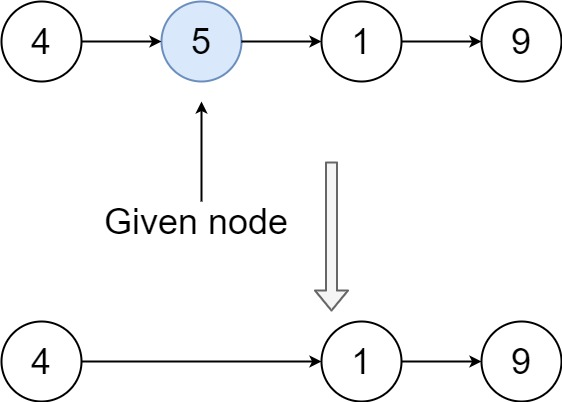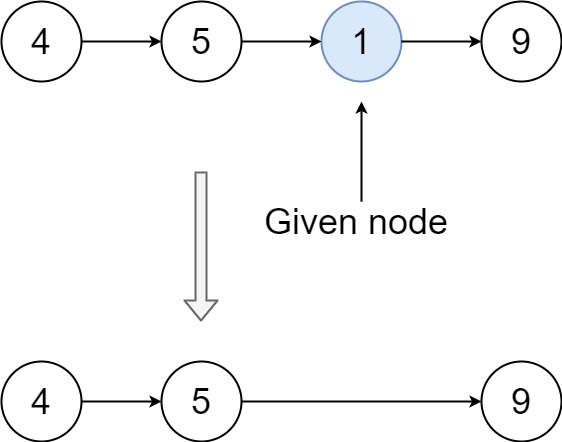# 237. Delete Node in a Linked List | Leetcode Solution

## 237. Delete Node in a Linked List

Write a function to delete a node in a singly-linked list. You will not be given access to the `head` of the list, instead, you will be given access to the node to be deleted directly.

It is guaranteed that the node to be deleted is not a tail node in the list.

Example 1:```Input: head = [4,5,1,9], node = 5
Output: [4,1,9]
Explanation: You are given the second node with value 5, the linked list should become 4 -> 1 -> 9 after calling your function.
```

Example 2:```Input: head = [4,5,1,9], node = 1
Output: [4,5,9]
Explanation: You are given the third node with value 1, the linked list should become 4 -> 5 -> 9 after calling your function.
```

Constraints:

• The number of the nodes in the given list is in the range `[2, 1000]`.
• `-1000 <= Node.val <= 1000`
• The value of each node in the list is unique.
• The `node` to be deleted is in the list and is not a tail node
The solution to the above question is-

/**
* struct ListNode {
*     int val;
*     ListNode *next;
*     ListNode(int x) : val(x), next(NULL) {}
* };
*/
class Solution {
public:
void deleteNode(ListNode* node) {
node->val=node->next->val;
if(node->next->next==NULL)
node->next=NULL;
else
node->next=node->next->next;

}
};